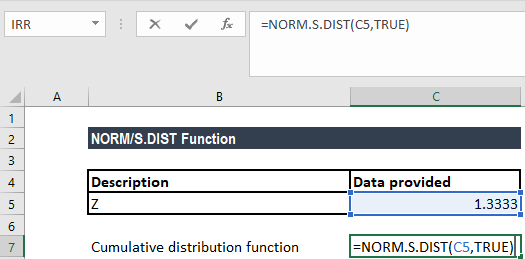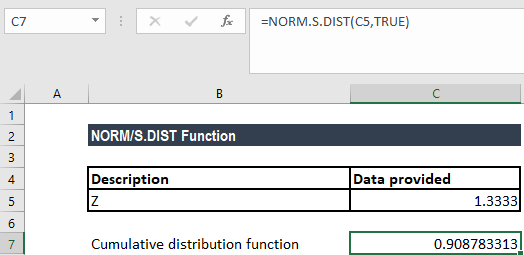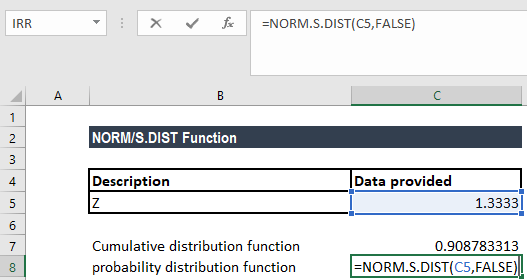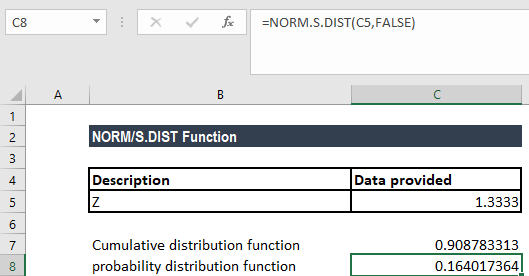# NORM.S.DIST Function

Get the standard normal distribution function for a given value

## How to Calculate Standard Normal Distribution

The NORM.S.DIST Function is categorized under Excel Statistical functions. It will calculate the Standard Normal Distribution function for a given value. The NORM.S.DIST function can be used to determine the probability that a random variable that is standard normally distributed would be less than 0.5.

In financial analysis, NORM.S.DIST helps calculate the probability of getting less than or equal to a specific value in a standard normal distribution. For example, it can be used for changes in the price indices, with stock prices assumed to be normally distributed.

### Formula

=NORM.S.DIST(z,cumulative)

The NORM.S.DIST function uses the following arguments:

1. Z (required argument) – This is the value for which we want the distribution.
2. Cumulative (required argument) This is the logical argument that denotes the type of distribution to be returned.

If “cumulative” = True (normal cumulative distribution function), then the area under the curve to the left of “x” is returned. That is, it would use the cumulative distribution function.

If “cumulative” = False (normal density function), the height of the curve at “x” is returned. That is, it would use the probability density function. So, the mean = 0 and the standard deviation = 1.

### How to use the Standard Normal Distribution Function in Excel?

To understand the uses of the NORM.S.DIST function, let’s consider an example of a standard normal distribution:

#### Example 1

Suppose we are given z = 1.333.

The formula used here for the cumulative distribution function is:We get the result below:The formula used for calculating the probability distribution function is:We get the result below:### A few notes about the NORM.S.DIST Function

1. The NORM.DIST() function refers to any normal distribution, whereas the NORMSDIST() compatibility function and the NORM.S.DIST() consistency function refer specifically to the unit normal distribution.
2. #VALUE! error – Occurs when any of the given arguments is non-numeric or is a non-logical value.
3. S.DIST is a special case of NORM.DIST. If we let the mean equal 0 and the standard deviation equal 1, the calculations for NORM.DIST match those of NORM.S.DIST.
4. The NORM.S.DIST function was introduced in MS Excel 2010 as a replacement for the NORMSDIST function.

Thanks for reading CFI’s guide to the Excel Standard Normal Distribution function. By taking the time to learn and master these functions, you’ll significantly speed up your financial analysis. To learn more, check out these additional CFI resources:

• Excel Finance Functions